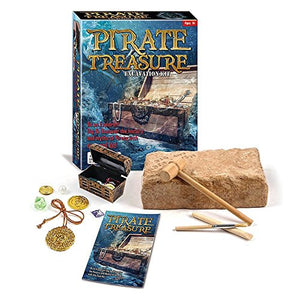# Pirate Treasure Chest Dig Excavation Kit

Vendor
DOMAGRON
Regular price
\$38.36
Sale price
\$27.88
Quantity must be 1 or more

• K

• i

• d

• s

• d

• i

• g

• t

• h

• i

• s

• e

• x

• t

• r

• a

• o

• r

• d

• i

• n

• a

• r

• y

• e

• x

• c

• a

• v

• a

• t

• i

• o

• n

• p

• r

• o

• j

• e

• c

• t

• .

• U

• s

• e

• t

• h

• e

• d

• i

• g

• g

• i

• n

• g

• t

• o

• o

• l

• t

• o

• c

• h

• i

• p

• a

• w

• a

• y

• a

• t

• t

• h

• e

• g

• y

• p

• s

• u

• m

• s

• a

• n

• d

• b

• l

• o

• c

• k

• t

• o

• u

• l

• t

• i

• m

• a

• t

• e

• f

• i

• n

• d

• a

• t

• h

• e

• p

• i

• r

• a

• t

• e

• t

• r

• e

• a

• s

• u

• r

• e

• .

• E

• a

• c

• h

• s

• e

• t

• i

• n

• c

• o

• l

• o

• r

• f

• u

• l

• p

• r

• i

• n

• t

• e

• d

• b

• o

• x

• .

• O

• f

• c

• o

• u

• r

• s

• e

• ,

• t

• h

• i

• s

• w

• i

• l

• l

• b

• e

• a

• t

• o

• y

• t

• r

• e

• a

• s

• u

• r

• e

• .

Kids dig this extraordinary excavation project. Use the digging tool to chip away at the gypsum sand block to ultimate find a the pirate treasure. Each set in colorful printed box. Of course, this will be a toy treasure.